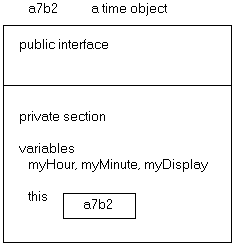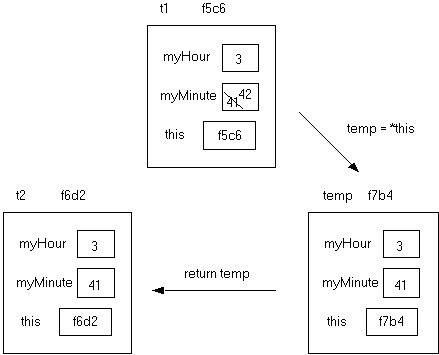Computer Science - C++

LESSON 30:  Classes, Abstraction, operator Overloading (Part III)

INTRODUCTION:

We now continue our development of the time and date class begun in Lesson 28 and further developed in Lesson 29.

The key topics for this lesson are:

A.  Pointer Variables and Dereferencing                        B.   The this Pointer

C.  Overloaded Increment Operators                            D.  Overloaded Relational Operators

VOCABULARY:

 POINTER THIS DEREFERENCING OPERATOR

DISCUSSION:                       A.  Pointer Variables and Dereferencing

1.   To understand usage of the this pointer with classes we need a brief introduction to pointer variables and the dereferencing operator.  (Note:  this will be covered more fully in Lesson 31.)

2.   A pointer variable stores a memory address.  The address operator (&) returns the address of a variable.  The syntax and usage of both of these new concepts is shown in Program 30-1.

Program 30-1

#include <iostream.h>

main()

{

int  num = 5;

int  *where;          // where is a pointer, stores an address

where = &num;    // where now stores the address of num

cout << "The address stored in where = " << where << endl;

cout << "The contents of *where = " << *where << endl;

return 0;

}

Run output (the address is machine-dependent):

The address stored in where = 222a95c

The contents of *where = 5

3.   The declaration  int  *where  implements a pointer variable.  The statement  where = &num  assigns the address of num to the pointer variable where.  The address stored in where is machine-dependent.

4.   The (*) operator is called the dereferencing operator.  This unary operator returns the contents of the address it is applied to.  The effect of  *where  is to return the contents of address 222a95c.  Another way to translate  *where  is to think of it as *(222a95c).  The first cout statement prints out the address stored in where, address 222a95c.  The second cout statement prints out the contents of *where, which is 5.

5.   Notice that the (*) operator is used in two different ways in this program.  When it is part of a variable declaration (int  *where) it is setting up a pointer variable.  When it is used as code (*where) it is dereferencing a memory address.  In this example the expression *where is an alias for num.

B.   The this Pointer

1.   When an object is instantiated, the object must reside in memory.  A program which declares an object will keep track of the starting address of this object.

2.   A C++ object maintains an internal reference to its own memory location.  This reference is stored as the this pointer.  The this pointer keeps track of the address of an instantiation of a class.  The time class we are developing comes with public member functions, its private variables, and a built-in private  this pointer.

Diagram 30-13.   The this pointer is a private identifier which can only be used by member functions within the class.  The this pointer is not accessible outside of the class.  In Diagram 30-1, this contains the starting address of the time object which contains this.  Each time object will contain its own this pointer, each holding a unique address where the object is stored in memory.

4.   We will use this when we overload the increment, decrement, and relational operators for the time class.

C.  Overloaded Increment Operators

1.   Here is a review of the results of the preincrement operator.  The statement  ++a  does two things.  It first adds one to the value of a, then returns the incremented value of a.  When this is used as a single line of code,

++a;

the value of a is one larger and the new value of a is returned.  In this case nothing is done with the returned value.  However in this code the return value is assigned to another variable:

int  a = 1, b;

b = ++a;

results in a = 2 and b = 2.

2.   The postincrement operator works differently.  The statement,

a++;

increments a one larger, then returns the old value of a.  The code:

int  a = 1, b;

b = a++;

results in a = 2 and b = 1.

3.   Overloading the preincrement and postincrement operators poses an unusual problem as the operator (++) is the same for both.  The C++ compiler will distinguish the two by the presence of an (int) signature for the postincrement operator.  Here are the member function prototypes for these two overloaded functions in the time class:

time operator++();       // preincrement

time operator++(int);  // postincrement

4.   The postincrement operator shows an (int) parameter, but it is strictly a notation to identify the postincrement operation.  An integer value is not passed.  Notice that both overloaded increment operators will return a time value as a value parameter.  It is the nature of this returned time value that differs for the two operators.

5.   Here is the implementation for the overloaded time preincrement operator:

time time::operator++ ()

{

if (59 == myMinute)

{

myMinute = 0;

if (23 == myHour)

myHour = 0;

else

myHour++;

}

else

myMinute++;

return *this;

}

Consider the declaration,

time t1;

which results in something similar to Diagram 30-2, which follows.  The memory address of object  t1  will be machine dependent.

Diagram 30-2When the expression  ++t1  is executed, the appropriate changes are made to myMinute and myHour.  The last statement  *this  at the end of the function is a dereferencing operation.  The function returns the contents of the memory address 2a9c, which is the time object t1.  When the function returns *this, it is returning a reference to itself, the time object t1.  However because the return type is a value parameter, the function returns a copy of the time object t1.

6.   The following code fragment illustrates the effect of using the preincrement operator in conjunction with the assignment operator:

time  t1 (3,26,'p');

time  t2;

t2 = ++t1;

The value of t1 is incremented by one minute and the operation ++t1 returns a copy of the object t1 to be assigned to t2.  The net effect is that both t1 and t2 equal 3:27 pm.

7.   The postincrement operator is implemented as:

// postincrement operation

time time::operator++(int)

{

time temp = *this;

++(*this);              // use the already defined preincrement operator

return temp;

}

The first step this function executes is to store a copy of the time object before it is changed.  The statement,

time  temp = *this;

dereferences the address of the time object and stores the contents of this address (the time object itself) in temp.  The incrementation is solved by calling the already defined preincrement operator.  After the incrementation is solved the function returns the time object stored in temp as a value parameter.  The code,

time  t1 (3,41,'p');

time  t2;

t2 = t1++;

results in assignments of values to three different time objects:  t1, t2, and temp.  In Diagram 30-3, which follows, the myDisplay field has been omitted from the diagrams although it is also assigned.  The sequence of events is:

1.   Do temp = *this

2.   Increment t1, the myMinute field changes from 41 to 42.

3.   Return temp as a copy to t2.

Diagram 30-3The resulting values stored in t1 and t2 after the statement

t2 = t1++;

are:  t1 = 3:42 pm  and  t2 = 3:41 pm.  The local value of temp is destroyed after the function is completed.

 See Handout H.A.28.1 time.h. 8.   The predecrement and postdecrement operators are implemented in similar fashion.  See Handout H.A.28.1 for the details.

D.  Overloaded Relational Operators

1.   Another valuable set of operations to support with time objects are relational operations:  <, >, ==, !=, >=, <=.  The function prototype for the less than (<) operator is given here:

bool time::operator< (const time &) const;  // overloaded < operator

It is appropriate to declare all of the relational operators as const   member functions.  This means that when this function is invoked, all private data members cannot be changed because it is a const member function.  It provides a measure of protection.

2.   Consider two time objects, t1 and t2 used in the following expression:         t1 < t2

This is interpreted as the function call:   t1.operator < (t2).

The private data members of the time object t1 will be compared with the private data members of time object t2.

3.   The implementation of the less than (<) function is:

bool time::operator< (const time &temp2) const

{

if (myHour < temp2.myHour)

return true;

else

if (myHour > temp2.myHour)

return false;

else  // myHour == temp2.myHour

return (myMinute < temp2.myMinute);

4.   The equality (==) relational operator is implemented as:

bool time::operator== (const time &temp2) const

{

return ((myHour == temp2.myHour) &&

(myMinute == temp2.myMinute));

}

5.   Once the less than (<) and equality (==) operators have been defined, the greater than (>) operator can be defined in terms of the other two member functions.  Recall the Boolean algebra logic,

if (x > 5) evaluates as true, then  not (x <= 5) must be true.

Within the implementation file of a class, member functions can be implemented in terms of other functions previously defined in the file.  Here is the implementation of the greater than (>) operator:

bool time::operator> (const time &temp2) const

{

return ! ((*this < temp2) || (*this == temp2));

}

The use of *this refers to the time object to which the member function belongs.  For example the code:   t1 > t2

is interpreted as the function call:     t1.operator > (t2).

Any reference to *this is actually an alias for the time object t1.  In the comparison (*this == temp2) we are actually comparing (t1 == t2).  Likewise (*this < temp2) is a comparison of

(t1 < t2).

6.   All of these functions use const reference parameters for maximum efficiency.

SUMMARY/REVIEW:         These last three lessons have been a major investment of time (!!!) and energy devoted to learning about classes.  The lab work will complete the date abstraction.

ASSIGNMENT:                    Lab Exercise, L.A.30.1, Date3

Lab Exercise, L.A.30.2, DaysApart